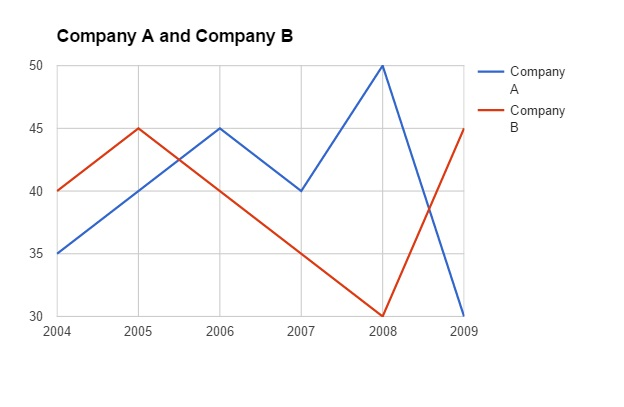## IBPS RRB Data Analysis Test 2

Instructions

Study the graph carefully to answer the questions that follow:
Per cent profit made by two companies over the years
Per cent profit=$$\frac{Income-Expenditure}{Expenditure}\times 100$$Q 1

If in the year 2004 the expenditures incurred by Company A and B were the same what was the ratio of the income of Company A to that company B in that year ?

Q 2

If the amount of profit earned by Company A in the year 2007 was Rs 1.5 lakhs what was its expenditure in that year ?

Q 3

What is the average per cent profit earned by Company B over all the years together ?

Q 4

If in the year 2008 the incomes of both the companies A and B were the same what was the ratio of the expenditure of Company B in that year ?

Q 5

What is the ratio of the amount of profit earned by Company A to that by company B in the year 2009?